# Special Theory Of Realativity NAT

## 10 Questions MCQ Test Topic wise Tests for IIT JAM Physics | Special Theory Of Realativity NAT

Description
This mock test of Special Theory Of Realativity NAT for Physics helps you for every Physics entrance exam. This contains 10 Multiple Choice Questions for Physics Special Theory Of Realativity NAT (mcq) to study with solutions a complete question bank. The solved questions answers in this Special Theory Of Realativity NAT quiz give you a good mix of easy questions and tough questions. Physics students definitely take this Special Theory Of Realativity NAT exercise for a better result in the exam. You can find other Special Theory Of Realativity NAT extra questions, long questions & short questions for Physics on EduRev as well by searching above.
*Answer can only contain numeric values
QUESTION: 1

### An observer O at rest midway between 2 sources of light at  x = 0 and  x = 10m, observes the 2 sources to flash simultaneously. According to a second observer O', moving at a constant speed parallel to the x-axis, one source flashes 13ns before the other. Find the speed of O' relatives to O (in units of c)

Solution:

A →  Flashing observed from first source (at x = 0)

B →​  Flashing observed from second source (at x = 10)

Now    Event (A) occurs (at x = 0)    Event B occurs (at x = 10)

For     t = 0    t = 0
For O'    t = 0     t = 13 ns

Here we assumed that both observers observe event A  at  t = 0

Now the problem says that for  O', one event occurs 13 ns  before the other.
∴   O' observes event B at  t = 13 ns
Now, applying transformation for time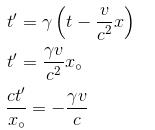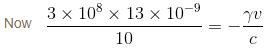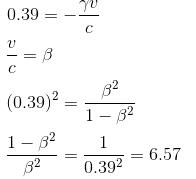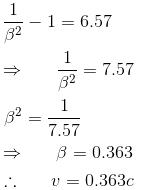The correct answer is: 0.363

*Answer can only contain numeric values
QUESTION: 2

### What is the speed of a particle having a momentum of 5MeV/c and a total relativistic energy of 10 MeV (in terms of c)?

Solution: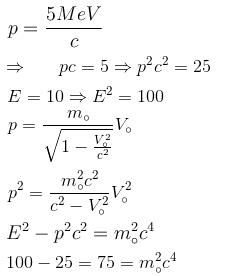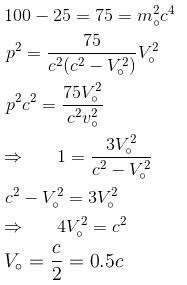The correct answer is: 0.5

*Answer can only contain numeric values
QUESTION: 3

### A tube of water is travelling at c/2 relative to the lab frame when a beam of light travelling in the same direction as the tube enters it. What is the speed of light in the water relative to the lab frame? (The index of water is 4/3)

Solution:

The beam of light travels through the water at a speed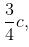while the tube itself is travelling at a speed c/2.

Using the addition of relativistic velocities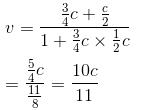= 0.909 c
The correct answer is: 0.909

*Answer can only contain numeric values
QUESTION: 4

A particle of mass M decays from rest into 2 particles. One particle has mass m  and the other particle is massless. Find the momentum of the massless particle (in units of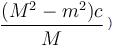Solution:

Momentum conservation

Pinitial = 0
Pfinal = mv + p  is (Here p is momentum of the massless particle)

mv + p = 0
p = –mv

Now, for the masses particle  E = p.  For the particle of mass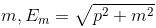from conservation of energy, [Take c = 1]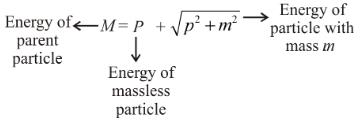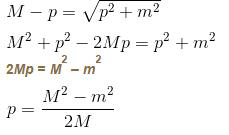∴   momentum of masses particle is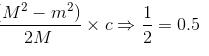The correct answer is: 0.5

*Answer can only contain numeric values
QUESTION: 5

The Lyman α spectral line of hydrogen (λ = 122nm) differs by 1.8 × 10–12 m  in spectra taken at the opposite ends of the sun’s equator. What is the speed of a particle on the equator due to the sun’s rotation, (in km/s)

Solution:

When the sun rotates, one end of the equator moves towards us & the other moves away from us.

So, the difference given is basically the difference between the red & blue shift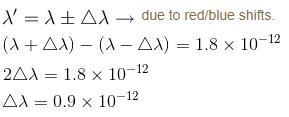Now, using the non relativisitic doppler effect (since the speed of sun is much less than C)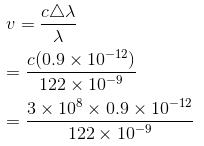v = 2.2 km/s
The correct answer is: 2.2

*Answer can only contain numeric values
QUESTION: 6

A distant galaxy is observed to have its hydrogen β line shifted to a wavelength of  580nm,  away from the lab value of 434nm.  Find the approximate velocity of the recession of the distant galaxy (in terms of c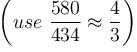Solution:

Original wavelength = 434 nm

shifted λ = 580 nm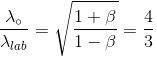(given in the problem)

Relativistic dopper effect formulae.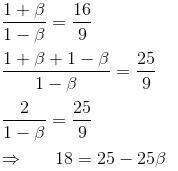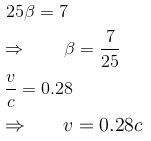= 0.28
The correct answer is: 0.28

*Answer can only contain numeric values
QUESTION: 7

Two space ships approach Earth with equal speeds as measured by an observer on Earth, but from opposite directions. A meter stick on one spaceship is measured to be 60 cm long by an occupant of the other ship. What is the speed of each spaceship as measured from earth. (in units of c)?

Solution: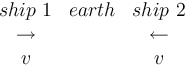length of stick on ship 1 measured by ship 2 = 60 cm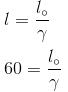Now, since each ship is moving with velocity v.

∴   Velocity of ship 2 wrt ship 1 = 2v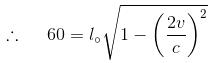l0 = 1m (meter stick)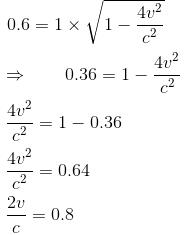v = 0.4c
Speed of each ship = 0.4c
The correct answer is: 0.4

*Answer can only contain numeric values
QUESTION: 8

In inertial frame S1 two events occur at the same time and 3c  minutes aparts in space. In the inertial frame S',  the same events occur at 5c  minutes apart. What is the time interval between the events in S' ? (in mins)

Solution:

In frame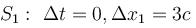In frame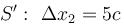Since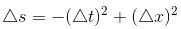is an invariant quantity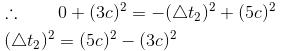= 25c2 – 9c2
= 16c2

Δt2 = 4c In frame S'

The correct answer is: 4

*Answer can only contain numeric values
QUESTION: 9

A photon strikes an electron of mass m that is initially at rest, creating an electron positron pair. The photon is destroyed and the positron and 2 electrons move off at equal speeds along the initial direction of the photon. Find energy of the photon (in units of  mc2).

Solution:

Let us work in the system of units in which c = 1

Now since E2 – p2  is an invariant quantity, we equate this in the initial laboratory frame and in the final frame of particles

In lab frame, the electron is at rest.
∴  Eelectron = m(c = 1) (Take m  to be the mass of electron)

∴  Total Energy of the system is E + m = Energy of photon + Energy of electron
Ep are the total energy and momentum of the photon.

∴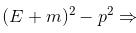Laboratory frame         ...(1)

In the final state of particles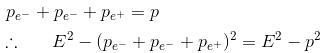E2 – p2 = (m + m + m)2
= (3m)2
= 9m2          ...(2)

Equating for the 2 frames  (1) = (2)
∴  (E + m)2 – p2 = 9m2
E2 + m2 + 2Em – p2 = 9m2
since a photon is massless

∴  E = p
∴  m2 + 2Em = 9m2
2Em = 8m2
E = 4m
Now in SI units
E = 4mc2

The correct answer is: 4

*Answer can only contain numeric values
QUESTION: 10

The half life of a π+ meson at rest is 2.5 × 10–8 s. A beam of  π+ mesons is generated at a point 15m  from a detector. Only half of the π+ mesons live to reach the detector. What is the speed of the π+ mesons? (in terms of c)

Solution: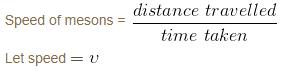In the rest frame of mesons,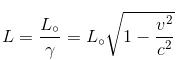Life time (in rest frame of mesons) = 2.5 × 10–8 s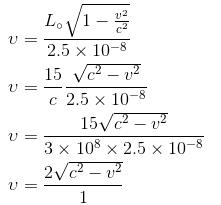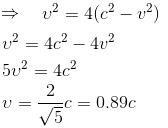= 0.89
The correct answer is: 0.89